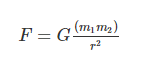We have studied science since elementary school and it is an important subject in academics. Chemistry, Biology, and Physics as the subcategories of science. Chemistry involves the study of chemicals, properties of the materials, compositions of chemicals, and chemical reactions. Biology is the scientific study of life where the structure of animals and plants is learned in detail. Various species of animals are studied along with the ecosystem and its conservation techniques.

Physics deals with pressure, volume, matter, friction, gravitation, temperature, and many more. Some laws like the Law of Universal Gravitation, Newton’s law, Pascal’s law, are the key knowledge to be known. In this article, we shall look into some fundamental concepts in Physics along with interesting laws. Physics is an interesting subject that has a connection with other disciplines of science. Its presence is seen in areas like quantum chemistry and biophysics.

Physics has evolved many years ago. The research and development by scientists and philosophers have significantly contributed to the advancement of the field. The foundation of these advancements is done on the basis of elementary concepts. So we can know that fundamental concepts are the basis for the milestones achieved.

Some of the basic concepts are energy, force, friction, motion, acceleration, mass, momentum, gravitational force, pressure, volume, and many more. Right from how we are able to walk, run, jump, and to the most interesting topic – the black hole depends on the concept of gravitation. It is the key force that holds galaxies, planets, stars, and is even responsible for the propagation of light. According to Newton’s Law of Universal Gravitation, it states that “Every particle attracts every other particle in the universe with a force that is directly proportional to the product of the masses and inversely proportional to the square of the distance between them”.

Universal gravitational equations are given bywhere F is the gravitational force between bodies, m1 and m2 are the masses of the bodies, r is the distance between the centers of two bodies, G is the universal gravitational constant.

The value of G = 6.673 x 10-11 N m2/kg2.

Now let us know about another elementary concept – pressure and understand what Pascal’s Law states.

### Pressure and Associated law

The physical force exerted on an object is known as pressure. Pressure can be exerted on solids, liquids, and gases as well. The SI unit of pressure is the pascal. When pressure is applied to a solid, it undergoes deformation. The solid may be crushed if the internal tensions exceed the material strength. The volume of the gas decreases when pressure is applied. Liquids are relatively incompressible than solids and gases. The increase in pressure at increasing depths in a liquid is known as liquid pressure. Pascals law states that “The external static pressure applied on a confined liquid is distributed or transmitted evenly throughout the liquid in all directions”.

Blaise Pascal was the French mathematician who stated Pascal’s Law. It explains that the static pressure acts at right angles to any surface in contact with the fluid. Pascal law is expressed using the formula F=PA.

Hope you have understood the concept of the Law of Universal Gravitation and Pascal’s Law along with its formula and SI unit.